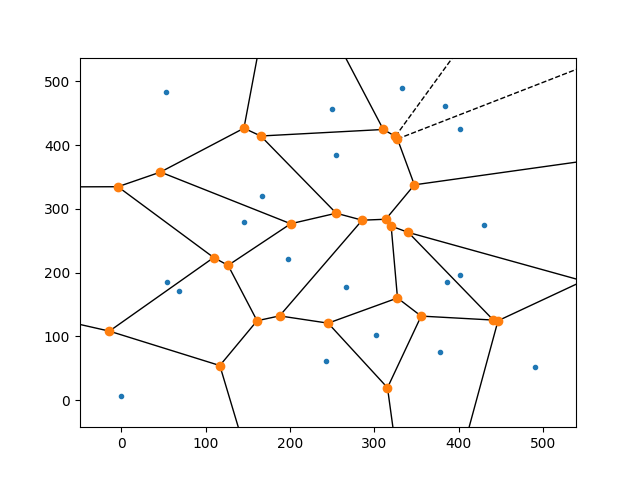# How can I get the vertices of each polygon of a Voronoi diagram?

39 views (last 30 days)
Eman S on 12 Jul 2018
Commented: Rena Berman on 14 May 2020 at 17:15
Hi all, For the following image;How can I get the vertices (which are highlighted by orange dots) of each Voronoi polygon of the following Voronoi diagram?
The picture is from the Internet. It is just for illustration.
Any suggestions for code to get these vertices?

KSSV on 13 Jul 2018
How you got this image? YOu plotted using inbuilt function? Or you have a image and you want to get points from the image?
Rena Berman on 14 May 2020 at 17:15

KSSV on 20 Jul 2018
x = gallery('uniformdata',[1 10],0);
y = gallery('uniformdata',[1 10],1);
[vx,vy] = voronoi(x,y);
plot(x,y,'r+',vx,vy,'b-')
axis equal
(vx,vy) are the vertices you want.

#### 1 Comment

Karishma q on 14 May 2019
How do I get the co-ordinates of each polygon separately? I tried adding a for loop which defines the number of regions, but I am still getting the vertices of the last region. Also [vx, vy]=voronoi(x,y) provides all the coordinates, I want for the individual regions. Can you help me on this?